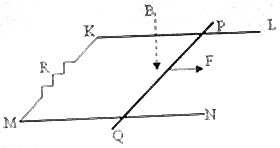## Pages

`“Life is like riding a bicycle.  To keep your balance you must keep moving.”–Albert Einstein`

## Friday, December 14, 2007

### AP Physics– Free Response Question (for practice) on Electromagnetic Induction

To build your confidence in answering free response questions, you may try the following practice question on electromagnetic induction:The adjoining figure shows two smooth, straight, conducting, horizontal, parallel rails KL and MN on which a straight conducting rod PQ of mass ‘m’ can slide without friction, in a direction parallel to the rails. The rails and the rod have negligible resistance; but, there is a resistance R connected between the rails at one end. A uniform magnetic field of flux density B tesla is applied vertically downwards, throughout the region. The separation between the rails is L1 and the length of the rod PQ is L2.
(a) The rod PQ is pulled along the rails by a constant horizontal force F applied parallel to the rails. Derive an expression for the terminal velocity (vterminal) of the rod.
(b) If the rod starts from rest with an initial acceleration ‘a1 ’, find its acceleration when the velocity of the rod is vterminal/4.
(c) What is the power dissipated in the resistance R in terms of the magnetic field and other known parameters, when the terminal velocity is attained?
(d) If the rod PQ is stationary and the magnetic field B is decreasing, will the direction of ihe induced current in the loop KPQM be clockwise or anticlockwise, when viewed along the direction of the magnetic field? Give reasons for your answer.
The above question carries 15 points which are divided among the parts (a), (b) and (c)and (d) as 6 + 5 + 2 + 2. Try to answer the above question within 15 minutes or less. I’ll be back with the answer shortly.